# Planetary Magical SquaresLogan Art/CreativeCommons

In the Western Occult Tradition, each planet has traditionally been associated with a series of numbers and particular organizations of those numbers. One such method of numerological arrangement is the magic square.

## Magic Square of Saturn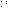Catherine Beyer

## Associated Numbers

The numbers associated with Saturn are 3, 9, 15 and 45. This is because:

• Each row and column of the magic square contains three numbers.
• The square contains nine numbers total, ranging from 1 to 9.
• Each row, column and diagonal adds up to 15.
• All of the numbers in the square add up to 45.

## Divine Names

The divine names associated with Saturn all have numerological values of 3, 9, or 15. The names of the intelligence of Saturn and the spirit of Saturn have a value of 45. These values are calculated by writing out the names in Hebrew and then adding up the value of each included letter, as each Hebrew letter can represent both a sound and a numerical value.

## Construction of The Seal

The seal of Saturn is constructed by drawing lines that intersect every number within the magic square.

## Magic Square of Jupiter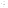Catherine Beyer

## Associated Numbers

The numbers associated with Jupiter are 4, 16, 34, and 136. This is because:

• Each row and column of the magic square contains four numbers.
• The square contains 16 numbers total, ranging from 1 to 16.
• Each row, column and diagonal adds up to 34.
• All of the numbers in the square add up to 136.

## Divine Names

The divine names associated with Jupiter all have numerological values of 4 or 34. The names of the intelligence of Jupiter and the spirit of Jupiter are 136. These values are calculated by writing out the names in Hebrew and then adding up the value of each included letter, as each Hebrew letter can represent both a sound and a numerical value.

## Construction of The Square

The square is constructed by first filling in each square with numbers 1 to 16 consecutively, starting at the bottom left with 1 and working upward toward the upper right with 16. Then specific pairs of numbers are inverted, i.e., they trade spaces. Opposite ends of the diagonals are inverted, as are the inner numbers on the diagonals, so that the following pairs are inverted: 1 and 16, 4 and 13, 7 and 10, and 11 and 6. The remaining numbers are not moved.

## Construction of The Seal

The seal of Jupiter is constructed by drawing lines that intersect every number within the magic square.

## Magic Square of Mars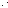Catherine Beyer

## Associated Numbers

The numbers associated with Mars are 5, 25, 65, and 325. This is because:

• Each row and column of the magic square contains five numbers.
• The square contains 25 numbers total, ranging from 1 to 25.
• Each row, column and diagonal adds up to 65.
• All of the numbers in the square add up to 325.

## Divine Names

The divine names associated with Mars all have numerological values of 5 or 65. The names of the intelligence of Mars and the spirit of Mars have a value of 325. These values are calculated by writing out the names in Hebrew and then adding up the value of each included letter, as each Hebrew letter can represent both a sound and a numerical value.

## Construction of The Square

The square is constructed by arranging the numbers consecutively in a pre-arranged pattern. In general, numbering moves down and to the right. Hence, 2 is down and to the right of 1. When the down and right motion would take you off the edge of the square, it wraps around. Thus, since 2 is on the bottom edge, 3 is still to the right of 2, but it's at the top of the square instead of the bottom.

When this pattern runs up against numbers already placed, the pattern shifts two rows down. Thus, 4 is on the left, 5 is one down and one to the right of 4, and if that motion were to be repeated, it would collide with the already placed 1. Instead, 6 appears two rows down from 5, and the pattern continues.

## Construction of The Seal

The seal of Mars is constructed by drawing lines that intersect every number within the magic square.

## Magic Square of the Sun (Sol)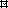Catherine Beyer

## Associated Numbers

The numbers associated with the Sun are 6, 36, 111, and 666. This is because:

• Each row and column of the magic square contains four numbers.
• The square contains 36 numbers total, ranging from 1 to 36.
• Each row, column and diagonal adds up to111.
• All of the numbers in the square add up to 666.

## Divine Names

The divine names associated with The Sun all have numerological values of 6 or 36. The name of the intelligence of the Sun has a value of 111 and the spirit of the Sun has a value of 666. These values are calculated by writing out the names in Hebrew and then adding up the value of each included letter, as each Hebrew letter can represent both a sound and a numerical value.

## Construction of The Square

The creation of the square of the Sun is messy. It is constructed by first filling in each square with numbers 1 to 36 consecutively, starting at the bottom left with 1 and working upward toward the upper right with 36. Numbers inside the boxes along the main diagonals of the square are then inverted, i.e., switch places. For example, 1 and 36 change places, as does 31 and 6.

Once this is done, more pairs of numbers still need to be inverted to make all of the rows and columns to add up to 111. There is no clean rule to follow to do such: it appears to have been done through trial and error.

## Construction of The Seal

The seal of the Sun is constructed by drawing lines that intersect every number within the magic square.

## Magic Square of Venus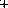Catherine Beyer

## Associated Numbers

The numbers associated with Venus are 7, 49, 175 and 1225. This is because:

• Each row and column of the magic square contains seven numbers.
• The square contains 49 numbers total, ranging from 1 to 49.
• Each row, column and diagonal adds up to 175.
• All of the numbers in the square add up to 1225.

## Divine Names

The name of the intelligence of Venus has a value if 49. The name of the spirit of Venus has a value of 175, and the name of the intelligence of Venus has a value of 1225. These values are calculated by writing out the names in Hebrew and then adding up the value of each included letter, as each Hebrew letter can represent both a sound and a numerical value.

## Construction of The Seal

The seal of Venus is constructed by drawing lines that intersect every number within the magic square.

## Magic Square of Mercury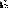Catherine Beyer

## Associated Numbers

The numbers associated with Mercury are 8, 64, 260, and 2080. This is because:

• Each row and column of the magic square contains eight numbers.
• The square contains 64 numbers total, ranging from 1 to 64.
• Each row, column and diagonal adds up to 260.
• All of the numbers in the square add up to 2080.

## Divine Names

The divine names associated with Mercury all have numerological values of 8 or 64. The name of the intelligence of Mercury has a value of 260, and the name of the spirit of Mercury has a value of 2080. These values are calculated by writing out the names in Hebrew and then adding up the value of each included letter, as each Hebrew letter can represent both a sound and a numerical value.

## Construction of The Seal

The seal of Mercury is constructed by drawing lines that intersect every number within the magic square.

Read more: More Correspondences of Mercury

## Magic Square of The Moon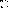Catherine Beyer

## Associated Numbers

The numbers associated with the Moon are 9, 81, 369, and 3321. This is because:

• Each row and column of the magic square contains nine numbers.
• The square contains 81 numbers total, ranging from 1 to 81.
• Each row, column and diagonal adds up to 369.
• All of the numbers in the square add up to 3321.

## Divine Names

The divine names associated with the Moon all have numerological values of 9 or 81. The name of the spirit of the moon has a value of 369. The names of the intelligency of the intelligence of the Moon and the spirit of the spirits of the Moon have a value of 3321. These values are calculated by writing out the names in Hebrew and then adding up the value of each included letter, as each Hebrew letter can represent both a sound and a numerical value.

## Construction of The Seal

The seal of the Moon is constructed by drawing lines that intersect every number within the magic square.

Read more: More Correspondences of The Moon
Format
mla apa chicago
Your Citation
Beyer, Catherine. "Planetary Magical Squares." Learn Religions, Feb. 8, 2021, learnreligions.com/planetary-magical-squares-4123077. Beyer, Catherine. (2021, February 8). Planetary Magical Squares. Retrieved from https://www.learnreligions.com/planetary-magical-squares-4123077 Beyer, Catherine. "Planetary Magical Squares." Learn Religions. https://www.learnreligions.com/planetary-magical-squares-4123077 (accessed April 18, 2021).